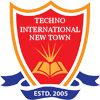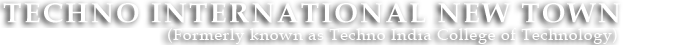#+91 9674112076/2079
••••# Teaching by finding solution of a real life engineering problem

In the first lecture I define a real life engineering problem and ask the students to suggest a solution. The selected problem is such that the solution requires the knowledge of the Course which I am going to teach. As a result, the students are unable to find any solution.After this I tell them that for the solution of the problem knowledge of the course are required. Also, I explain the problem in detail and establish a layout for solution based on the course content. This exercise increases their interest for the course. After completion of each module I discuss with the students how the content of the module contribute to the partial solution of the problem.
Take Power Electronics (EE603) as an example. I am teaching this course to the 3rd year electrical engineering students. The problem I defined is “How to develop a home inverter “. I explained the problem and solution as follows.

1. The different parts of home inverter are AC-DC converter, battery charge controller (DC-DC converter), and DC-AC converter (PWM voltage source Inverter).
2. The power electronics switches are required to develop the converters & inverters.
3. Their semiconductor physics, V-I & gate characteristics, protection & gate triggering scheme of the devices & circuits.
4. Topology, operating principle, control scheme of the converters & inverters.

It can be easily found that the above topics are covering the major syllabus of the course. My experience with this strategy is inspiring.